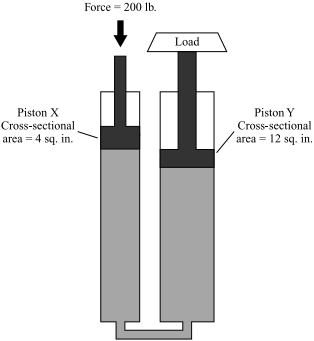Massachusetts Comprehensive Assessment System

# 2013, High School Technology/Engineering

Reporting Category: Fluid and Thermal Systems
Standard: 3.3 - Calculate and describe the ability of a hydraulic system to multiply distance, multiply force, and effect directional change.

A hydraulic system is represented below.A force of 200 lb. is applied to piston X and a load is placed on piston Y.
1. Calculate the pressure exerted by piston X on the hydraulic fluid. Show your calculations and include units in your answer.
2. What is the pressure that the hydraulic fluid exerts on piston Y? Explain your answer.
4. If piston X moves down 3 in., what distance does piston Y move up? Include units in your answer.

## Scoring Guide and Sample Student Work

Select a score point in the table below to view the sample student response.

ScoreDescription
4 The response demonstrates a thorough understanding of the relationship between force, pressure, and motion in a hydraulic system. The response correctly calculates the pressure exerted by piston X. The response also correctly identifies the pressure on piston Y, explains the answer, calculates the force exerted on piston Y to support the load, and finds the distance that piston Y moves up for a given movement of piston X.
4
3
The response demonstrates a general understanding of the relationship between force, pressure, and motion in a hydraulic system.
2
The response demonstrates a limited understanding of the relationship between force, pressure, and motion in a hydraulic system.
1
The response demonstrates a minimal understanding of the relationship between force, pressure, and motion in a hydraulic system.
0 Response is incorrect or contains some correct work that is irrelevant to the skill or concept being measured.
Note: There are 2 sample student responses for Score Point 4.

## High School Technology/Engineering

Question 11: Construction and Manufacturing
Question 23: Fluid and Thermal Systems
Question 33: Fluid and Thermal Systems
Question 44: Electrical and Communication Systems
Question 45: Engineering Design

Last Updated: August 11, 2022